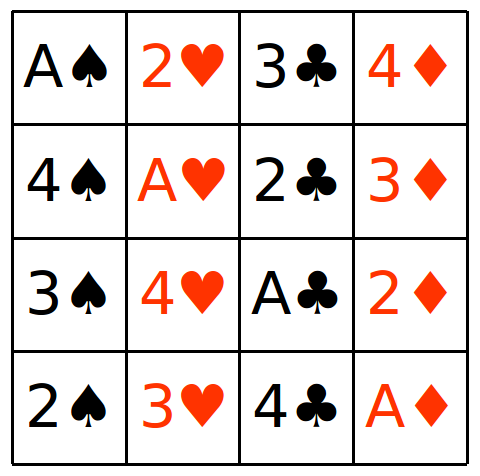# Magic Squares from Latin Squares

The most recent game I uploaded to this site is a Printable Latin Square Puzzle. The goal of the puzzle is to arrange a set of 16 cards into a 4 by 4 grid, following certain rules.

On the puzzle page, I provide some downloadable printable cards. You could use them, but cards from an ordinary pack of playing cards will do. You only need sixteen cards, for example, all the aces, twos, threes and fours, four from each suit.

When you’ve solved the grid, each row, column and diagonal, and some other blocks of four, will have one card of each number, and one of each suit. It’s like a miniature Sudoku puzzle on steroids.

Here’s an example of a failed attempt to solve the puzzle. It’s not a solution because the columns don’t have one of each suit:

However, each row is okay. Each row has

• An Ace, a two, a three and a four
• A spade, a club, a diamond and a heart.

We can turn this into a grid of numbers simply by allocating points for each suit and number. For example,

• I’ll say Aces are 1, twos are 2, threes are 3, and fours are 4.
• I’ll also say Spades are 0, Clubs are 4, Hearts are 8 and Diamonds are 16.

For example, the 4 of hearts is worth 12 points. Then, my grid will look like this:

Notice that:

• All the numbers from 1 to 16 appear in the grid.
• Each row adds up to 34.

The rows add up to 34 because each row has exactly one each of the numbers A, 2, 3 and 4, for a total of 1+2+3+4 = 10 points, and also one each of the suits spades, clubs, hearts, and diamonds, for a total of 0+4+8+12 = 24 points. That’s 34 points total in each row.

If I’d actually found a proper solution to the Latin Squares puzzle, and converted it to numbers, every row, column and diagonal, and a whole bunch of other blocks of four cells, would also add up to 34. I’d have a magic square!

I didn’t have to allocate points exactly the way I did here. As long as one group of “things” (suits or numbers) gets 1,2,3 and 4 points allocated to them, and the other group of things gets 0,4,8 and 12 points, a solution to the Latin Squares puzzle will give you a magic square.

But not vice-versa! There are many 4×4 magic squares that don’t come from solutions to this puzzle!